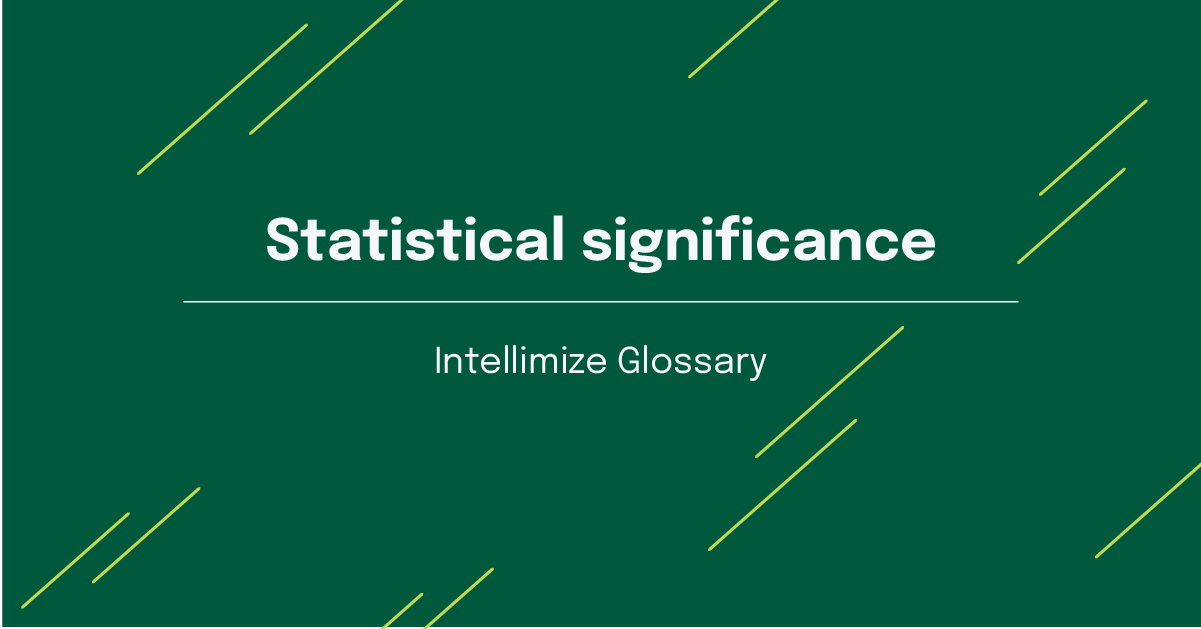All Resources
Glossary

# Statistical significance

#### Mar 06, 2023## What Is Statistical Significance?

Statistical significance (stat sig) is a metric in statistical analysis that supports the validity of a given result. Statistical significance essentially says, "How likely are the results i'm seeing NOT explained solely by chance or random factors." The higher the value, the more likely the results shown are "real" and not a coincidence.

Put into context of a marketing campaign, statistical significance shows whether visitor engagement with a brand’s content was a direct result of campaign efforts or whether similar results would have occurred in the absence of that campaign. In other words, "Are the results you're seeing truly caused by your efforts and not by something unexpected and random that would invalidate the interpretation of the results?"

## Why Is Statistical Significance Important for CRO?

It's important to know if your test results are valid so that you can rely on the results going forward as you further refine your website optimization strategy. Are your prospects actually interested in this type of content? If so, they'll likely be receptive to it and similarly themed content. If the results were caused by chance, however, and you invest a lot of effort and money in similar material, it has the risk of being a flop.

For certain experiments, like in A/B testing, statistical significance gives you the confidence that you need to act on the test results. High statistical significance means that the results of the experiment were not reached randomly but instead were produced because the variables that were part of the experiment influenced each other. In other words, the campaign was a success.

Employing statistical significance as part of website testing ensures that you’re making informed, high-value decisions to deliver a greater return on investment.

## How Is Statistical Significance Calculated?

To calculate the statistical significance of a campaign, marketing teams first must define their hypothesis to learn what statistical significance would look like for a given experiment. An experiment testing for statistical significance would encompass the following steps.

1. Define a hypothesis. Any experiment should start with a research-based hypothesis, or an educated guess about what the experiment will prove or disprove.
1. Start collecting data. Once you know what you want to test, you can start setting parameters for the tests. For example, you can decide when you want to begin the test, how long you’d like to run it, and how many variations you plan to test.
1. Ensure a large-enough sample size. In order for statistical significance to even be possible in an experiment, the experiment must contain a large enough sample size. After all, the more data points you have to measure, the more accurate the analysis will be.
1. Determine the significance level. Also known as alpha (α) or the threshold, the significance level indicates the level of risk of a Type 1 error, also known as a false positive. The significance should be as close to zero as possible in order to achieve the most accurate results. For most experiments, the risk level is usually 0.05, which means there is a 5% chance of a Type 1 error.
1. Find the p-value. In a website testing experiment, the p-value is the probability that the test will produce an observed result at the same level or greater than the results gathered from the data. The smaller the p-value, the more likely there is an alternative hypothesis. Finding the p-value means the experiment has ended and there is enough information to confirm whether statistical significance has been reached.
1. Calculate the results. Once the above steps have been completed, experimenters can calculate statistical significance using the following formula:

Probability (p) < Threshold (α) = Statistical significance

## How Is Statistical Significance Applied in Website Testing?

Statistical significance models can help marketing teams optimize multiple activities, including A/B testing.

### A/B testing

Statistical significance can be used to improve A/B testing efforts, particularly through tests that are meant to determine email clicks, open rates, engagements, landing page conversion rates, customer browsing behaviors, reactions to product launches, and calls-to-action (CTAs) on a given web page.

A/B testing requires a null hypothesis, which states that the experiment will not result in any significant findings. Experimenters also should come up with an alternative hypothesis, which is the hypothesis the experiment is intended to prove.

These types of experiments also require thresholds, or significance levels, that will describe how valid your theory is. Setting this number before the experiment will result in more conclusive results.

### Multivariate testing

In multivariate testing, multiple variables are modified as part of an experiment to determine which combinations of these variations perform as intended. It’s not as easy to reach statistical significance with multivariate testing as it is with A/B testing, but not reaching statistical significance with multivariate testing does not mean your tests are not reliable.

However, experimenters must closely inspect early indicators of success in the test rather than relying on statistical significance to inform decisions about a website optimization strategy. For example, there may be outliers that indicate certain creative elements aren’t resulting in success, which may mean these creative elements require their own tests.

Recommended content: